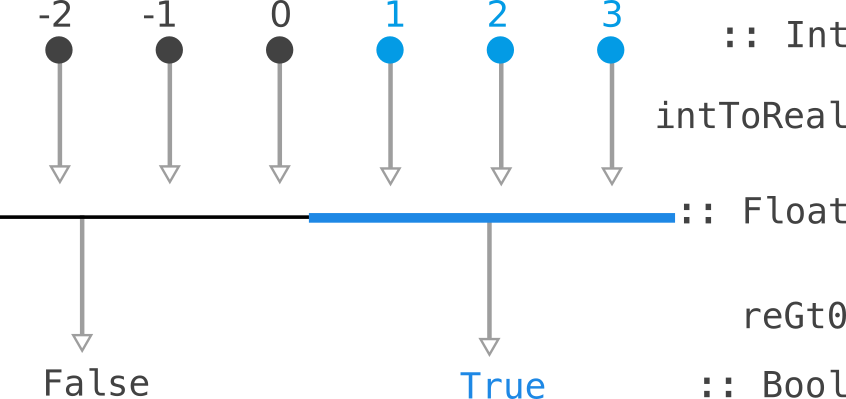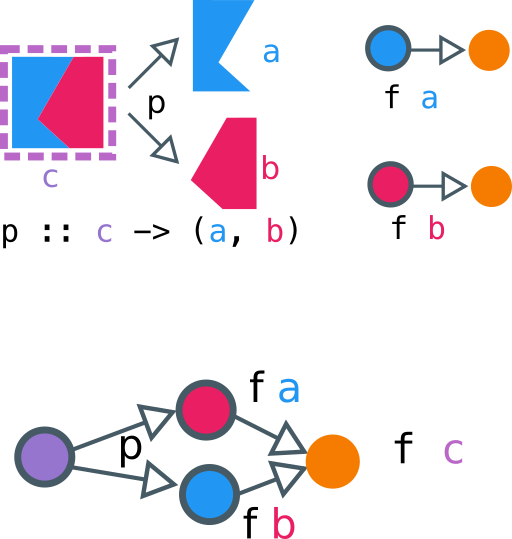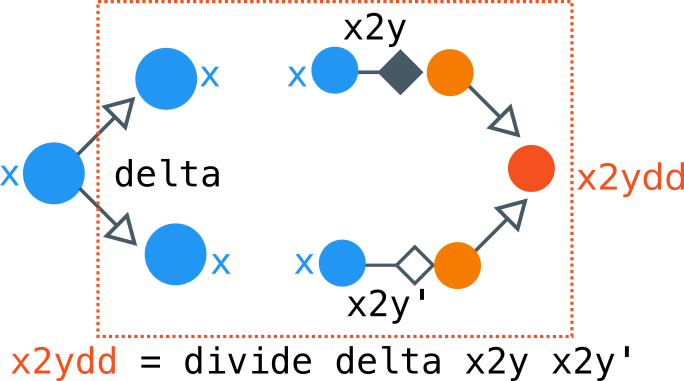# Through the Looking Class: Contravariant Functors and Applicatives

Siddharth Bhat

16 Mar 2021In this blog post, we will learn about `Contravariant` and `Divisible` which provide duals for `Data.Functor` and `Data.Applicative` respectively.

``````{-# LANGUAGE NoImplicitPrelude #-}
{-# LANGUAGE InstanceSigs #-}
import GHC.Base hiding (Functor)
import GHC.Float -- for Ord instance of Float
import GHC.Show -- for show
``````

## Dual Functors

First, a quick recap of functors:

``````class Functor f where
fmap :: (a -> b) -> f a -> f b
``````

This lets us lift a function `f: a -> b` into a `fmap f: f a -> f b`. The dual is called `Contravariant`:

``````class Contravariant f where
contramap :: (a -> b) -> f b -> f a
``````

Let us look at some example to build our intuition of such a typeclass.

## Predicates

The classic example is that of a predicate, which is something that tells us whether a value of type `t` obeys some property or not:

``````data Predicate t = Predicate { runPredicate :: t -> Bool }
instance Contravariant Predicate where
contramap :: (a -> b)
-> Predicate b -- b -> Bool
-> Predicate a -- a -> Bool
contramap a2b (Predicate b2bool) =
Predicate (\a -> b2bool (a2b a))
``````

An example of such a thing is if we know how to check a real number is greater than zero:

``````reGt0 :: Predicate Float
reGt0 = Predicate (\x -> x > 0.0)
``````

and we can converts integers into reals:

``````intToReal :: Int -> Float
intToReal i = error "TODO" -- fromIntegral
``````

then we can check if an integer is greater than zero:

``````intGt0 :: Predicate Int
intGt0 = contramap intToReal reGt0
``````

This is described by the picture:So, such a `Predicate Float` "consumes" a `Float` to produce a `Bool`. We can pull back the consumption along a function `Int -> Float` to consume a `Int` and produce a `Bool`.

## Dual Applicatives

``````class Functor f => Applicative f where
pure :: a -> f a
(<*>) :: f (a -> b) -> f a -> f b
``````

Recall that an `Applicative` allow us to work with tuples:

``````liftA2 :: (a -> b -> c) -> f a -> f b -> f c
``````

We can write the type of liftA2 to be more suggestive as:

``````liftA2 :: ((a, b) -> c) -> ((f a, f b) -> f c)
``````

If we can combine a tuple `(a, b)` into a value `c`, then we can glue lifted values `(f a, f b)` into a lifted `f c`.

The dual, called `Divisible`, says that if we can break a value `c` into `(a, b)`, then we can glue lifted values `(f a, f b)` into a lifted `f c`.

``````class Contravariant f => Divisible f where
divide :: (c -> (a, b)) -> f a -> f b -> f c
conquer :: f a
``````

The `conquer` is some sort of "default procedure" we can perform for any value. It'll be something benign, as we'll see when we check out the examples.Above, we have a picture of how to think about `Divisible`. The box with pink-and-blue is a `c`, that contains an `a` and a `b`. We have a function `p` that shows us how to split a `c` into an `a` and a `b`. We also have `f a` and `f b`, which consume `a, b` to produce some orange output. If we have this data, we can build an `f c`, something that can consume a `c`, by (1) splitting `c` into `(a, b)`, and then consuming the `(a, b)` using `f a` and `f b`.

## Example 1: Predicates

We can continue our example of predicates. If we know how to check if something holds for `a` and something holds for `b`, we can check how something holds for `(a, b)`: check for both `a` and `b`. So, this would be:

``````instance Divisible Predicate where
divide :: (c -> (a, b)) ->
Predicate a -> Predicate b -> Predicate c
divide c2ab (Predicate a2bool) (Predicate b2bool) =
Predicate (\c -> let (a, b) = c2ab c
in a2bool a && b2bool b)
``````

As for when we know nothing, we could either allow it or disallow it. In this case, since we are `&&` ing information, the way to be "benign" is to allow things (that is, return a `True`). Since `True && b = b`, we are sure that the `conquer` is indeed benign.

``````  conquer :: Predicate a
conquer = Predicate (\a -> True)
``````

## Example 2: Serialization

Consider the ability to convert a data type to a string. These "consume" the (varying) data types to produce a `String`. So, for example:

``````data Serializer a = Serializer { serialize :: a -> String }
``````

If we know how to print an `b` (that is, we have `b2string :: b-> String`), and we can turn `a`'s into `b`s, we compose the two to print `a`s:

``````instance Contravariant Serializer where
contramap :: (a -> b) -> Serializer b -> Serializer a
contramap a2b (Serializer b2string) =
Serializer (\a -> b2string (a2b a))
``````

For our `Divisible` instance, if we can print `a` and `b`, and we can break a `c` into an `(a, b)`, we (1) break the `c` down, and then (2) print the `a` and the `b`, and (3) concatenate the string representation of `a` and `b`:

``````instance Divisible Serializer where
divide :: (c -> (a, b))
-> Serializer a
-> Serializer b
-> Serializer c
divide c2ab (Serializer a2str) (Serializer b2str) =
Serializer (\c -> let (a, b) = c2ab c
in (a2str a) <> (b2str b))
``````

As for `conquer`, if we don't know how to print something, the best thing to do is to not print anything at all. This prevents us from garbling output. Thus, the benign choice for `conquer` is to print an empty string:

``````  conquer :: Serializer a
conquer = Serializer (\a -> "")
``````

We can put `Serializer` work immediately. For example, say we know how to serializer `Int`s and `Float`s:

``````intSerial :: Serializer Int
intSerial = Serializer (\i -> show i)

floatSerial :: Serializer Float
floatSerial = Serializer (\f -> show f)
``````

If we now have a type that contains `Int` and `Float`, no problem! `Divisible` has our back to combine the `Serializer`s together:

``````data Foo = Foo Int Float
fooSerial :: Serializer Foo
fooSerial = divide (\(Foo i f) -> (i, f))
intSerial floatSerial
``````

## Example 3 / Generalization: Fixed output type

We can generalize both examples: we have seen before: `Predicate` is all functions into a fixed output type `Bool`, while `Serializer` is functions into a fixed output type `String`. We need to know how to combine the outputs --- in the case of `Bool`, we combined the outputs with `&&`. In the case of `String`, we combined the outputs with `<>`. In general, we need a monoid.

``````data Into y x = Into { runInto :: x -> y }
instance Contravariant (Into y) where
contramap :: (b -> a)
-> Into y a -- a -> y
-> Into y b -- b -> y
contramap b2a (Into a2y) =
Into (\b -> a2y (b2a b))
``````

For the `divide`, we combine the data from `a` and `b` using the monoid of `y`:

``````instance Monoid y => Divisible (Into y) where
divide :: (c -> (a, b))
-> Into y a -- a -> y
-> Into y b -- b -> y
-> Into y c -- c -> y
divide c2ab (Into a2y) (Into b2y) =
Into (\c -> let (a, b) = c2ab c
in (a2y a) <> (b2y b))
``````

For conquer, the "benign instance" is the `mempty` value of the monoid, which by definition does not "interact" with any element, as `mempty <> m = m` and `m <> mempty = m`:

``````   conquer :: Into y a -- a -> y
conquer = Into (\a -> mempty)
``````

In all of these examples, we have (a) A data structure that can be decomposed: this is the part of `c -> (a, b)`, and (b) A consumer of data: `f a` is "something that can consume an `a`.

## The laws for Contravariant

So far, I have been skating on intuition, without telling you what the laws `Divisible` must follow are. Let's get formal now. For a given `Contravariant f`, we need a `fmap`-like law to hold:

• `fmap`'s law:: `fmap (f . g) = fmap f . fmap g
• `contramap`'s law: `contramap (f . g) = contramap g . contramap f`

See that the order gets flipped in comparison to `fmap`. Let us check that this law holds for `Into y`, since that was the most general example.

``````contramap :: (p -> q) -> Into y q -> Into y p
x2q :: x -> q
contramap (x2q . p2x) \$ (Into q2y) =?=
contramap p2x . conramap x2q \$ (Into q2y)
``````

We can rewrite our `Into y` definition to be easier to manipulate using point-free style:

``````instance Contravariant Into where
contramap :: (b -> a)
-> Into a -- a -> y
-> Into b -- b -> y
contramap b2a (Into a2y) = Into (a2y . b2a) -- b -> y
``````

if we now try to simplify:

1. `contramap p2x . contramap x2q \$ (Into q2y)`
2. Remove `.` and `\$`: `contramap p2x (contramap x2q (Into q2y))`
3. unwrap inner `contramap`: `contramap p2x (Into (q2y . x2q))
4. unwrap outer `contramap`: `Into (q2y . x2q . p2x)`
5. re-group `.`: `contramap`: `Into (q2y . (x2q . p2x))`
6. introduce back `contramap`: `contramap (x2q . p2x) (Into q2y)`

thus we are done! We've shown that the `Contravariant` laws hold for `Into`

## The laws for Divisible

The laws follow from some category theory. We need that for the function:

``````delta :: a -> (a, a)
delta a = (a, a)
``````

the following relations between `divide` and `conquer` hold:

1. First, let us think about `divide delta`. It means that we perform the same action on the left element and right element of the tuple since our tuple is built from the same element `a`.``````dd :: Divisible f => f a -> f a -> f a
dd = divide  delta
```

1. `conquer` is an identity element for `divide delta`:

```hs
dd m conquer = dd conquer m
```

2. `divide delta` is associative:

```hs
dd m (dd n o) = dd (dd m n) o
```

So this is saying that `divide delta` is monoidal, with `conquer`
as the identity element. Let's verify what happens in our case of `Into y`.

0. Let `a2y, a2y' :: Into y a`.
1. Expand the definition: `dd  (a2y, a2y') = divide delta (a2y, a2y')`
2. Expand `divide`:

```hs
divide delta a2y, a2y'
= Into (\c -> let (a, b) = delta c
in (a2y a) <> (a2y' b))
```
3. Substitue `delta c = (c, c)`

```hs
divide delta a2y, a2y'
= Into (\c -> let (a, b) = (c, c)
in (a2y a) <> (a2y' b))
```

4. Replace `a, b` with `c`

```
divide delta a2y, a2y' = Into (\c -> (a2y c) <> (a2y' c))
```

Great, so we have a simple enough definition of what `dd` does; It runs
both `a2y` and `a2y'` ou the same input and smashes the results. At this
point, it should be hopefully _somewhat_ clear why the laws hold for `Into`:

1. We build `conquer` using `mempty`, Since `mempty` is the identity for `(<>)`,
`conquer should be the identity for `divide delta`.
2. We are smashing outputs using `(<>)` in `divide delta`. As `(<>)` is associative, we should get

![divide-delta-conquer.png](https://functionalworks\-backend\-\-prod.s3.amazonaws.com/logos/51e8bfabb350afa67eec66d52ea2b4ac)

Pictorially, we are combining two machines: one that turns `x2y`, and one that is `conquer` which is "useless". Since we start by copying `x` using `delta x = (x, x)`, whatever `conquer` does is useless, and then only effect that's leftover is whatever the `x2y` does. So we can simplify the above figure by eliminating the bottom part of the computation, leaving us with this:

![divide-delta-conquer-simplify.png](https://functionalworks\-backend\-\-prod.s3.amazonaws.com/logos/bf5f27875bd4848699b6b28c07d20250)

``````

Siddharth Bhat

mathematics ⋂ computation

See other articles by Siddharth

Related jobs

See all

### WorksHub

CareersCompaniesSitemapFunctional WorksBlockchain WorksJavaScript WorksAI WorksGolang WorksJava WorksPython WorksRemote Works

### For companies

hello@works-hub.com

Ground Floor, Verse Building, 18 Brunswick Place, London, N1 6DZ

108 E 16th Street, New York, NY 10003